# 动态规划 求最大连续子数组、Python range 函数指南、Postman 导出 curl 命令、AWS 知识图谱大赛架构设计、John 易筋 ARTS 打卡 Week 26John(易筋)

# 题目

Given an integer array nums, find the contiguous subarray (containing at least one number) which has the largest sum and return its sum.

Follow up: If you have figured out the O(n) solution, try coding another solution using the divide and conquer approach, which is more subtle.

Example 1:

Input: nums = [-2,1,-3,4,-1,2,1,-5,4]Output: 6

Explanation: [4,-1,2,1] has the largest sum = 6.

Example 2:

Input: nums = Output: 1

Example 3:

Input: nums = Output: 0

Example 4:

Input: nums = [-1]Output: -1

Example 5:

Input: nums = [-2147483647]Output: -2147483647

Constraints:

1 <= nums.length <= 2 * 104-2^31 <= nums[i] <= 2^31 - 1

# 动态规划解法

class MaxmumSubarray:    def maxSubArray(self, nums: List[int]) -> int:        if nums == None or len(nums) == 0:            return 0        for i in range(1, len(nums)):            if nums[i - 1] > 0:                nums[i] += nums[i - 1]        return max(nums)

# 分而治之解法

class MaxmumSubarray:    def maxSubArray(self, nums: List[int]) -> int:        return self.helper(nums, 0, len(nums) - 1)    def helper(self, nums: List[int], left: int, right: int) -> int:        if left >= right:            return nums[left]        mid = (int)((left + right) / 2)        leftSub = self.helper(nums, left, mid)        rightSub = self.helper(nums, mid+1, right)        leftMax = nums[mid]        rightMax = nums[mid + 1]        temp = 0        for i in range(mid, left - 1, -1):            temp += nums[i]            if temp > leftMax:                leftMax = temp        temp = 0        for k in range(mid+1, right + 1):            temp += nums[k]            if temp > rightMax:                rightMax = temp        return max(max(leftSub, rightSub), leftMax + rightMax)

# 说明

range 当您需要执行特定次数的操作时，Python 的内置函数非常方便。作为一个经验丰富的 Pythonista，您很可能曾经使用过它。但是它是做什么的呢？

• 了解 Pythonrange 函数的工作原理

• 了解 Python 2 和 Python 3 中的实现方式有何不同

• 看到了许多动手的 range()例子

• 有能力克服一些局限性

• 让我们开始吧！

# 让我们循环

captains = ['Janeway', 'Picard', 'Sisko']for captain in captains:    print(captain)

JanewayPicardSisko

numbers_divisible_by_three = [3, 6, 9, 12, 15]for num in numbers_divisible_by_three:    quotient = num / 3    print(f"{num} divided by 3 is {int(quotient)}.")

3 divided by 3 is 1.6 divided by 3 is 2.9 divided by 3 is 3.12 divided by 3 is 4.15 divided by 3 is 5.

# Pythonrange()基础

for i in range(3, 16, 3):    quotient = i / 3    print(f"{i} divided by 3 is {int(quotient)}.")

>注意：尽管此示例显示了的适当用法 range()，但 range()在 for 循环中使用它的频率通常过高。

captains = ['Janeway', 'Picard', 'Sisko']for i in range(len(captains)):    print(captains[i])

range()这对于创建数字的可迭代变量非常有用，但是当您需要对可能被 in 运算符循环的数据进行迭代时，这并不是最佳选择。

1. range(stop) 有一个论点。

2. range(start, stop) 有两个参数。

3. range(start, stop, step) 需要三个参数。

## 1. range(stop)

for i in range(3):    print(i)

012

## 2. range(start, stop)

for i in range(1, 8):    print(i)

1234567

# 3. range(start, stop, step)

>>> range(1, 4, 0)Traceback (most recent call last):  File "<stdin>", line 1, in <module>ValueError: range() arg 3 must not be zero

for i in range(3, 16, 3):    quotient = i / 3    print(f"{i} divided by 3 is {int(quotient)}.")

numbers_divisible_by_three：3 divided by 3 is 1.6 divided by 3 is 2.9 divided by 3 is 3.12 divided by 3 is 4.15 divided by 3 is 5.

# 随着增加 range()

for i in range(3, 100, 25):    print(i)

3285378

# 递减 range()

for i in range(10, -6, -2):    print(i)

1086420-2-4

for i in reversed(range(5)):    print(i)

43210

range()使得可以对递减的数字序列进行迭代，而 reversed()通常用于以相反的顺序循环序列。

# Python range()函数的高级用法示例

range() 主要用于两个目的：

1. 执行 for 循环的主体特定次数

2. 比使用列表或元组创建更有效的整数可迭代

range() 是 Python 中的一种类型：

>>> type(range(3))<class 'range'>

>>> range(3)1>>> range(3)2

>>> range(6)[2:5]range(2, 5)

# 浮游物语

• 是整数

• 不包含小数点

• 可以是正数，负数或 0

• 可以是任何包含小数点的数字

• 可以是正数或负数

for i in range(3.3):    print(i)

Traceback (most recent call last):  File "<stdin>", line 1, in <module>TypeError: 'float' object cannot be interpreted as an integer

# range()与 NumPy 一起使用

NumPy是第三方 Python 库。如果要使用 NumPy，则第一步是检查是否已安装它。

>>> import numpy

import numpy as npnp.arange(0.3, 1.6, 0.3)

array([0.3, 0.6, 0.9, 1.2, 1.5])

import numpy as npfor i in np.arange(0.3, 1.6, 0.3):    print(i)

0.30.60.89999999999999991.21.5

# 说明

Postman 导出 curl 命令的步骤：

# 1. 请求链接：点击 Code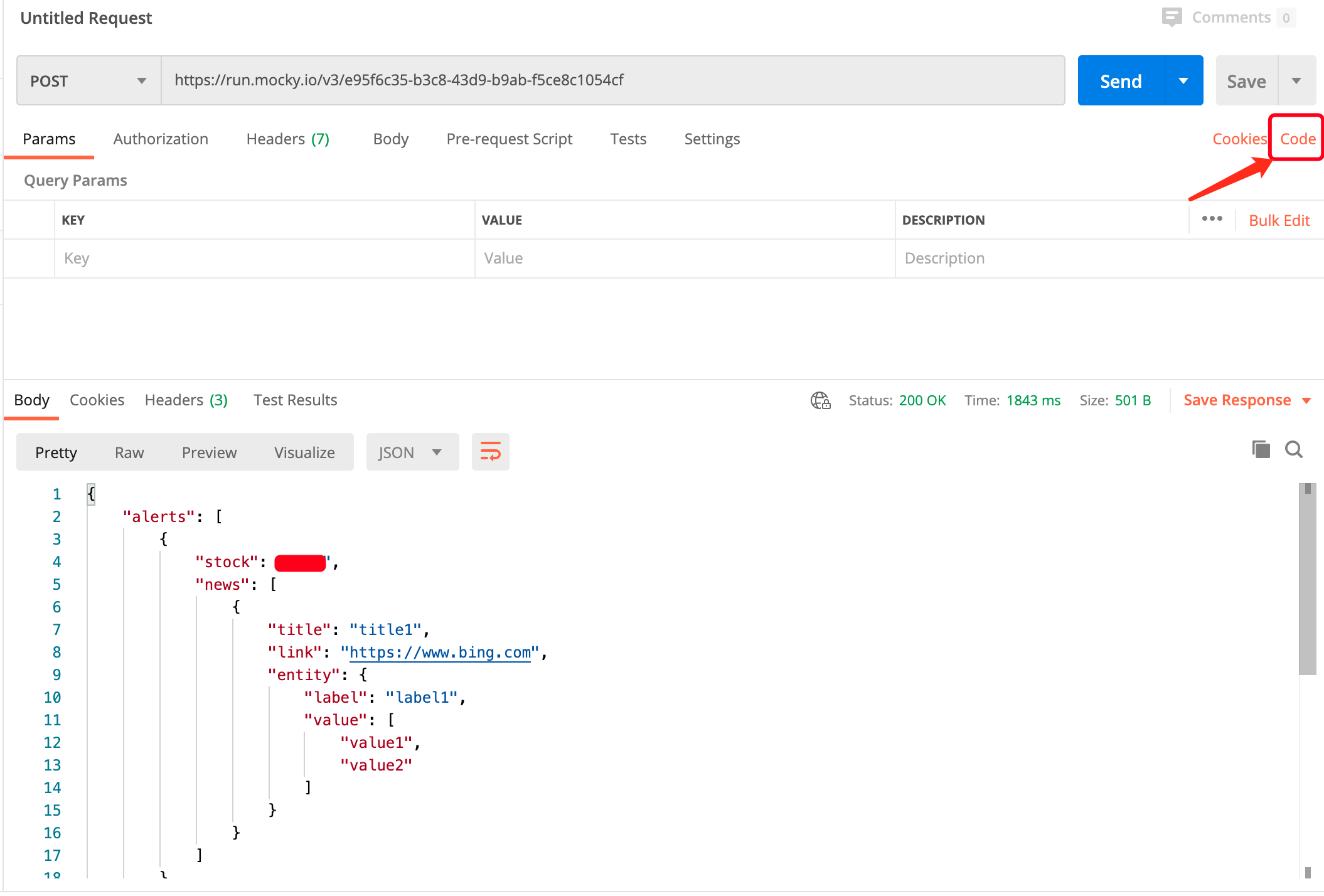# 2. copy cURL 的请求链接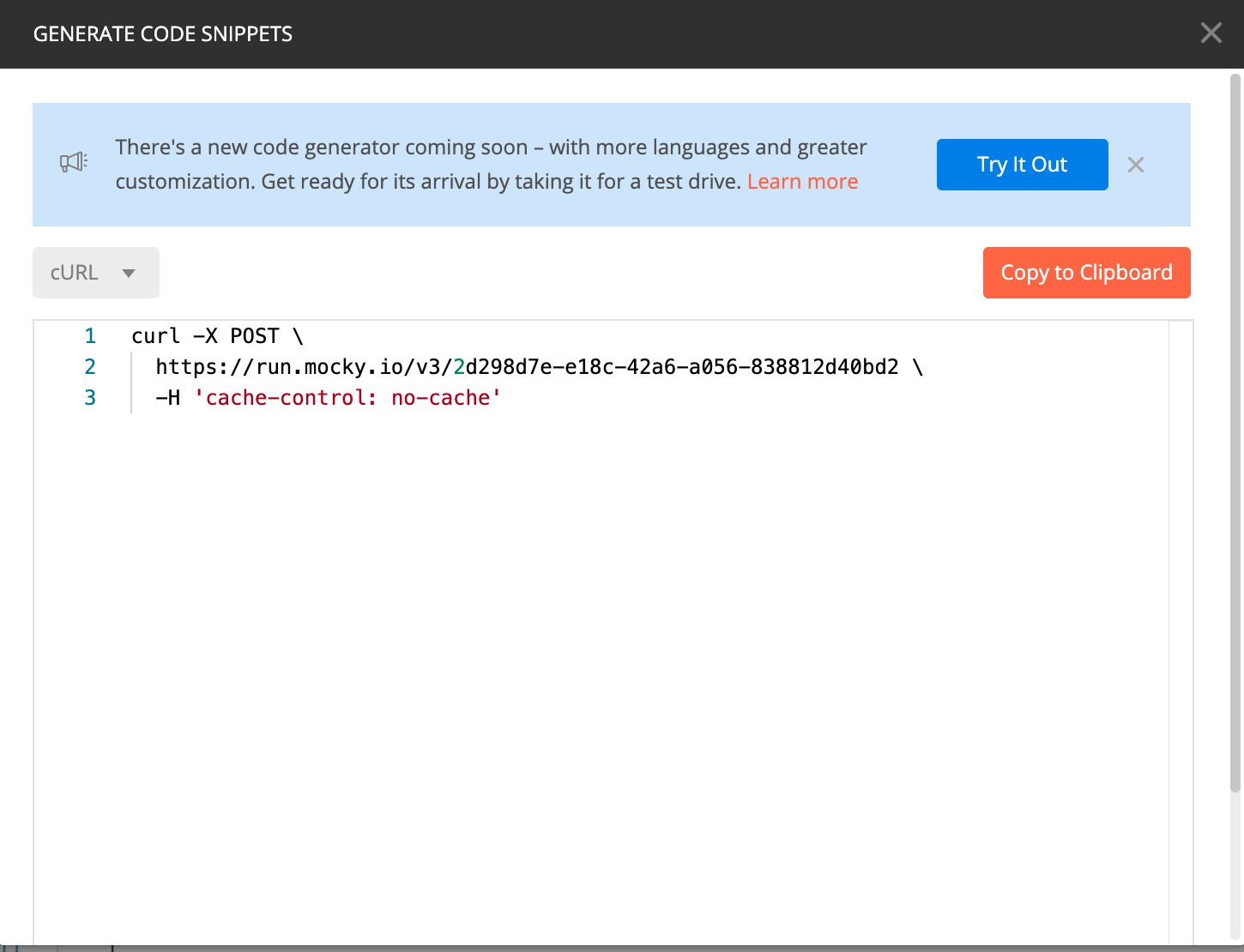# 3. 导出 curl 到 Terminal 运行

curl -X POST \  https://run.mocky.io/v3/e95f6c35-b3c8-43d9-b9ab-f5ce8c1054cf \  -H 'cache-control: no-cache'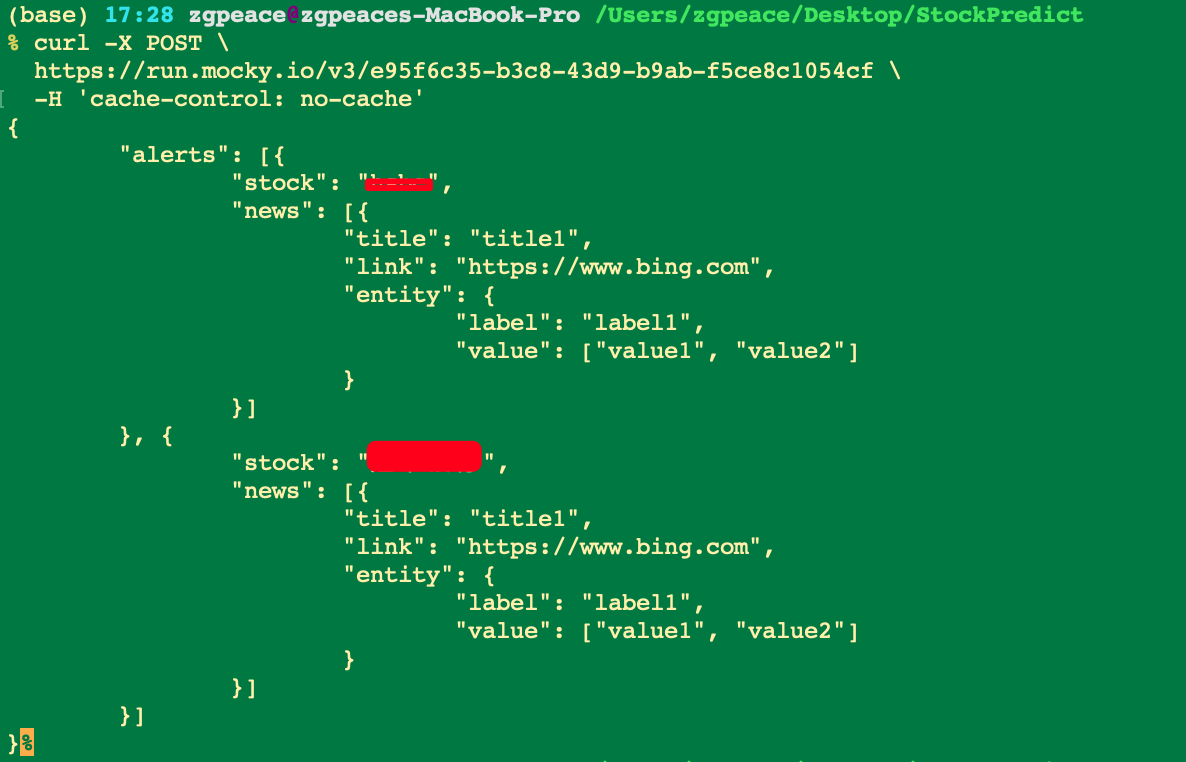# 1 设计概述

## 1.1 功能概述

### 1.1.1 数据获取以及存储场景如下：

a) 获取新闻数据的渠道：爬虫系统、海知(第三方公司)提供、通联(第三方公司)提供。

b) 获取股票数据的渠道：通联(第三方公司)提供。

c) 分析系统对新闻数据、股票进 NLP 分析，比如对新闻数据进行情感分析，分析出负面新闻。

d) 图数据库 Neptune 导入数据(格式 CSV)，新闻数据、股票数据；

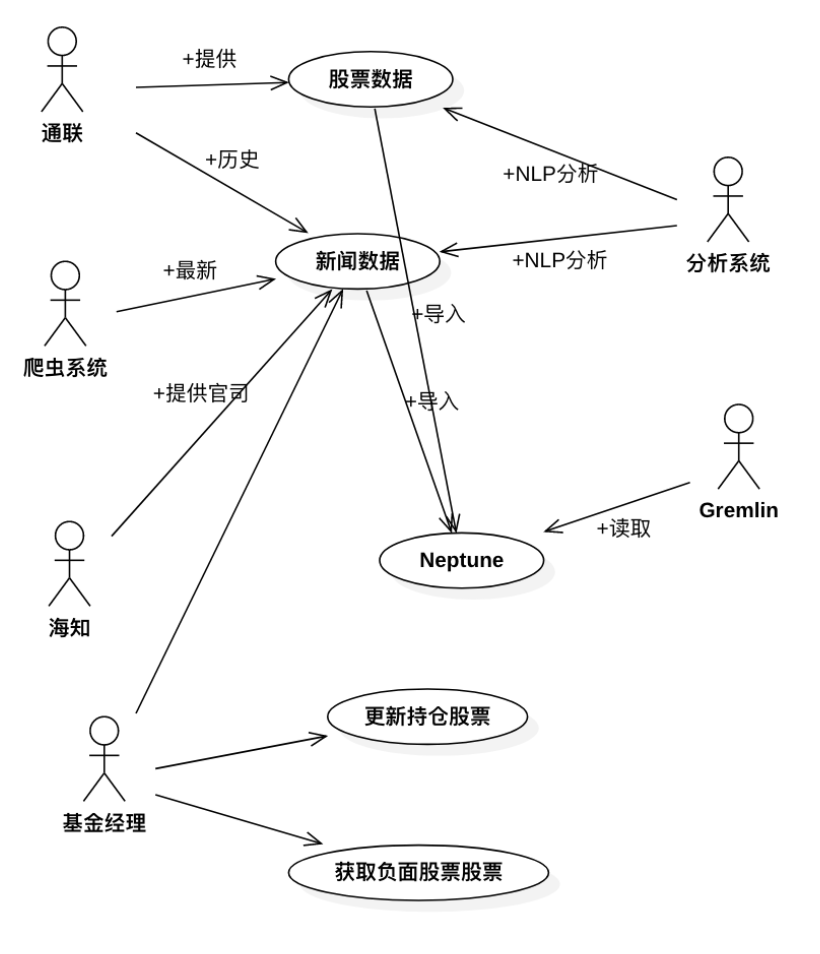### 1.1.2 训练模型以及预测用例图：

1)SageMaker 训练模型；

2)部署模型系统；

3)预测股票基金走势；

4)预测结果与实际结果进行对比，多维度打分；

5)重复上面的步骤，优化模型，提升预测分数。

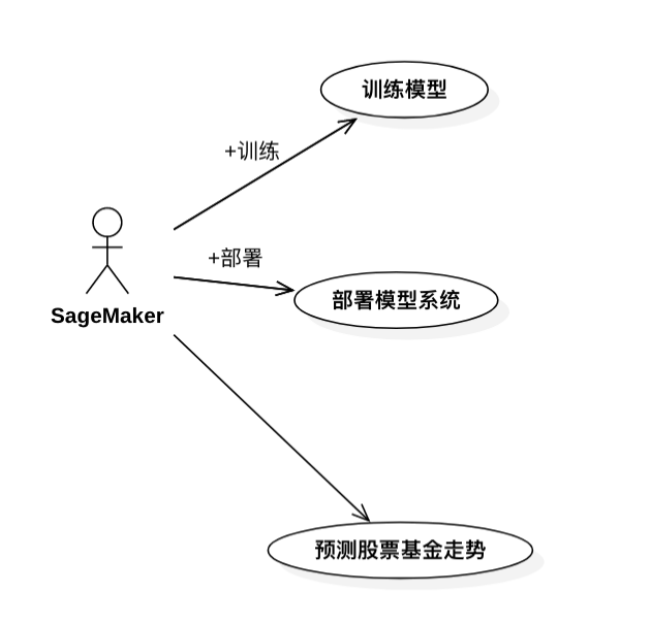## 1.2 ⾮功能约束

1.查询性能⽬标：平均响应时间<300m s，95% 响应时间<500m s，单机 T PS>100；

2.监控性能⽬标：平均响应时间<800m s，95% 响应时间<1000m s，单机 T PS>30；

3.系统核⼼功能可⽤性⽬标：>99.99% ；

4.系统安全性⽬标：系统可拦截 DDDOS 攻击，密码数据散列加密，客户端数据 HT T PS 加密，外部系统间通信对称加密；

5.数据持久化⽬标：>99.999% 。

## 1.3 用户流程## 1.4 产品概况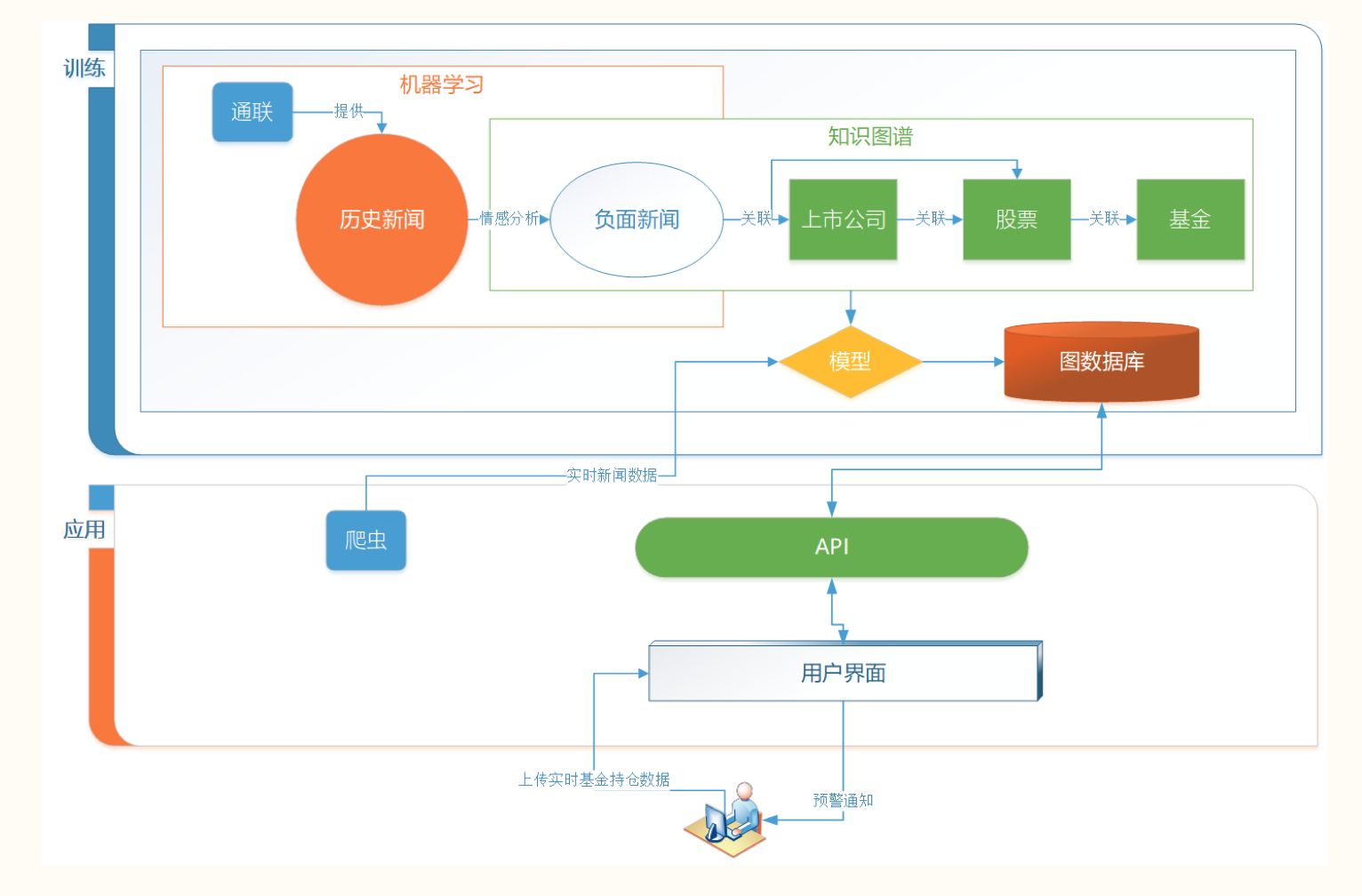# 2. 系统部署图与整体设计

## 2.1 知识图谱架构图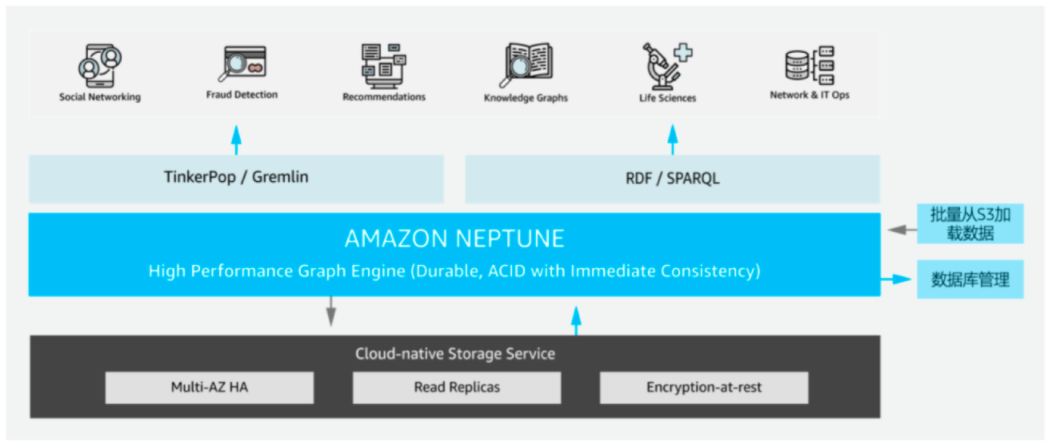

## 2.2 数据展示 时序图

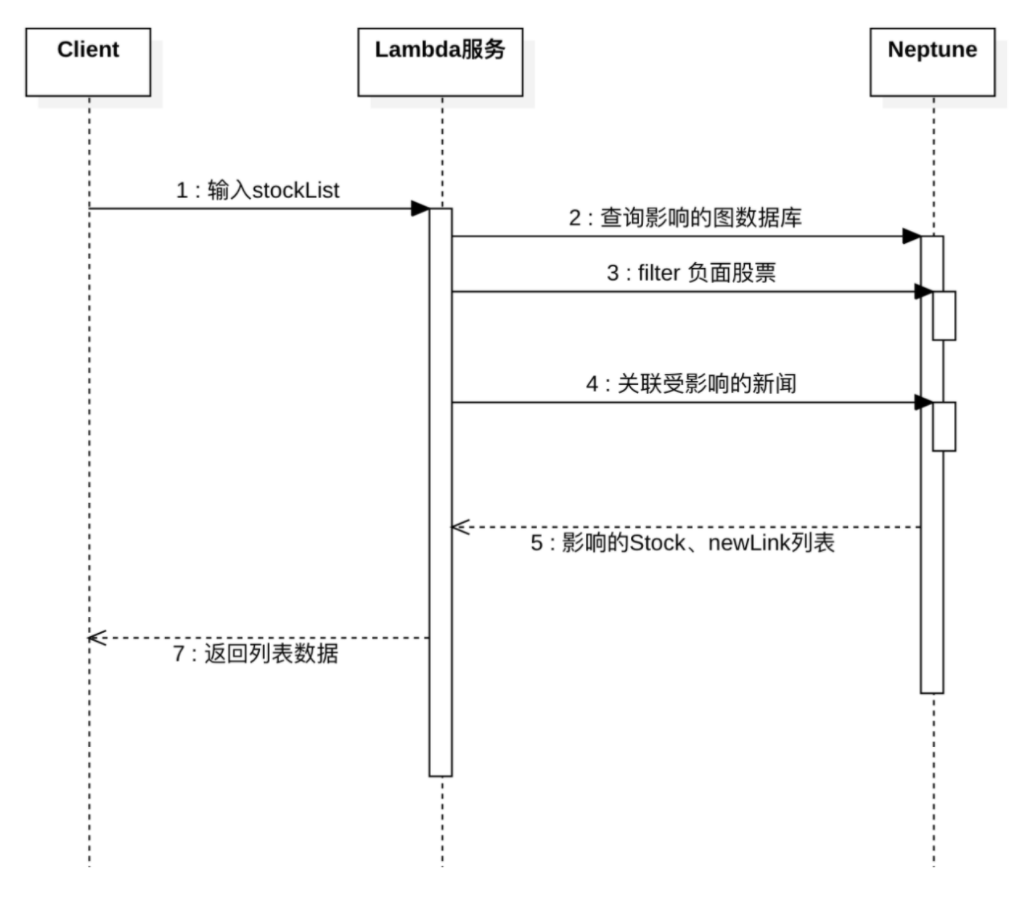## 2.3 新闻数据获取、以及导入图数据库 Neptune 场景系统序列图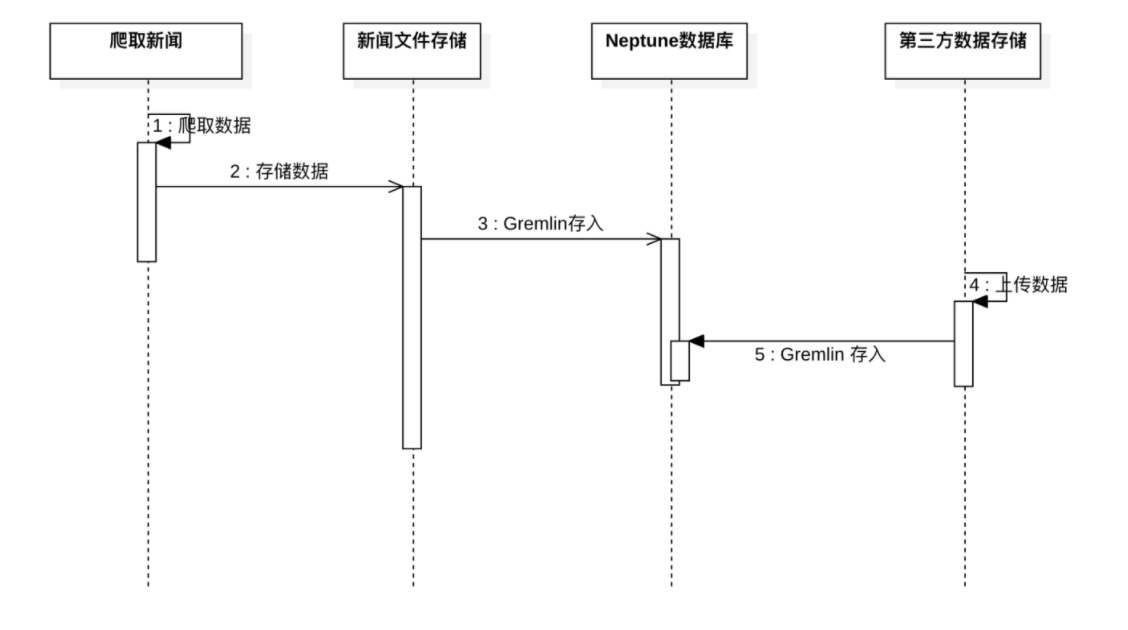

## 2.4 模型训练及模型应用预测趋势时序图

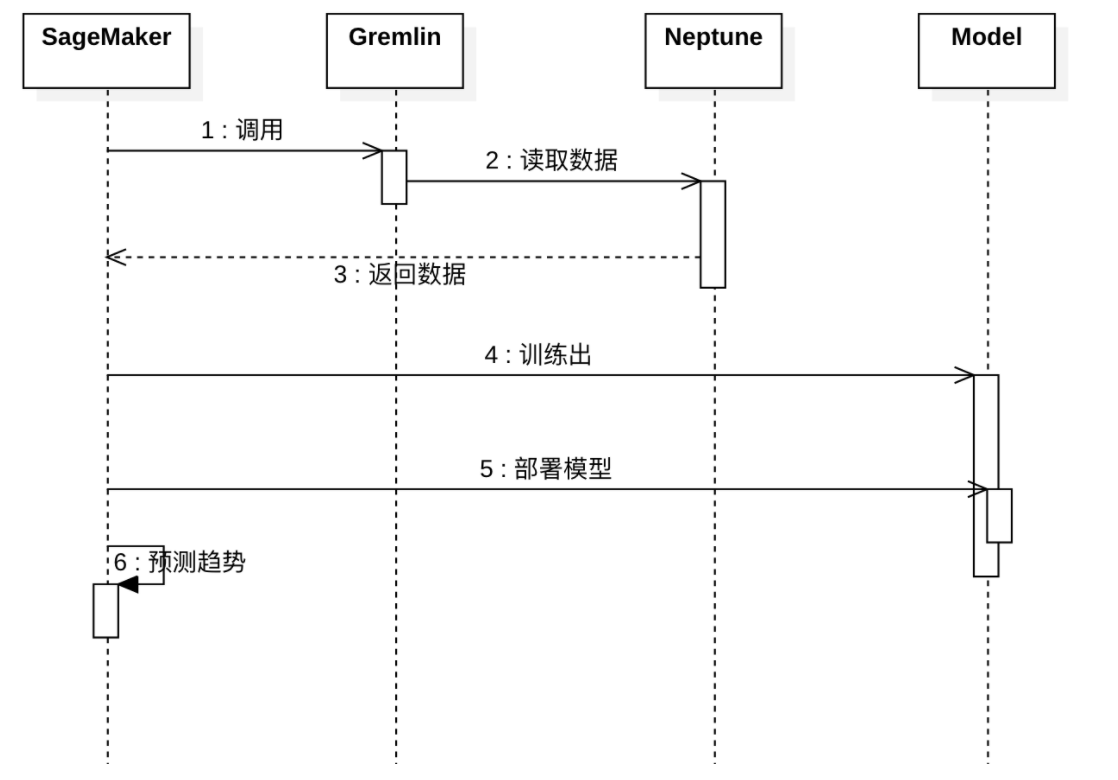# 3. 训练模型流程图 -- 负面新闻影响股票基金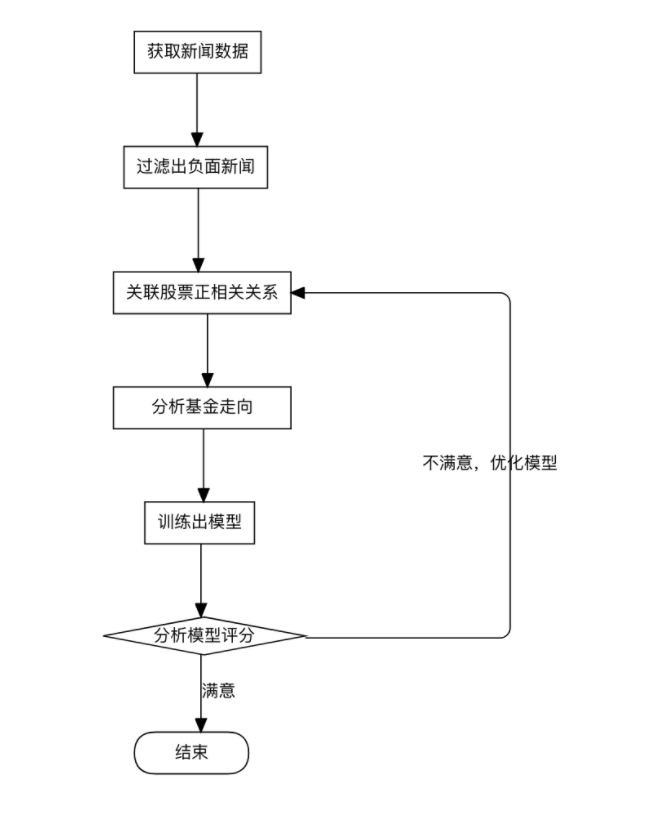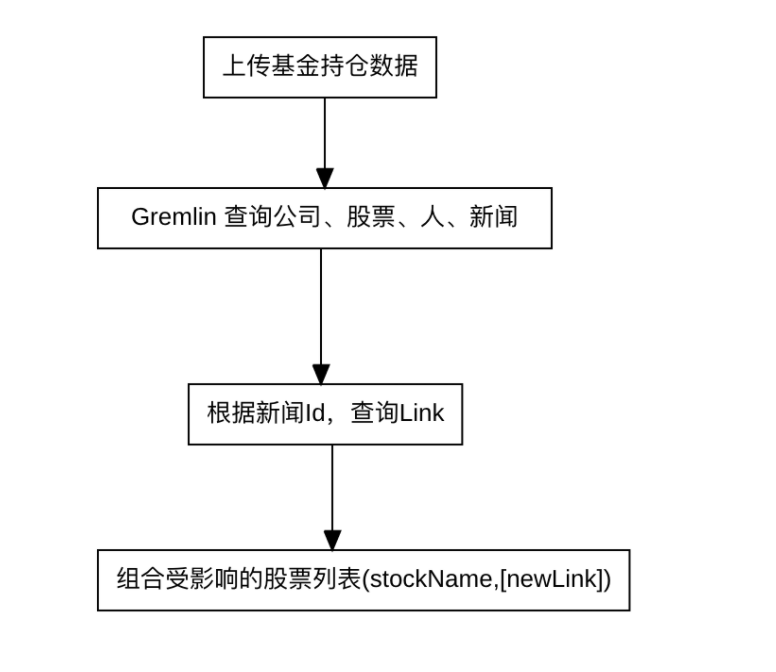

## 3.1 模型状态图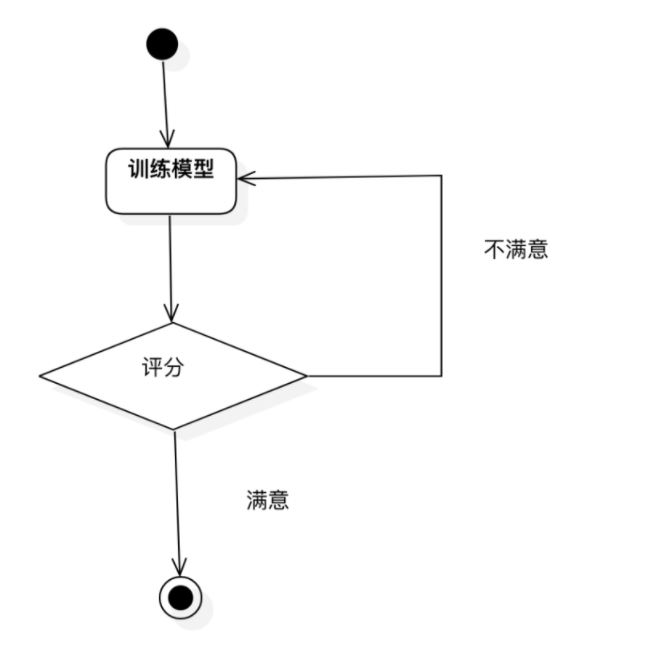

a) 训练模型>评分满意或者不能继续优化 > 训练结束。

b) 训练模型>评分不满意> 回到上一步继续优化。

1. 正向和负面新闻是否有抵消作用，还是参考 PageRank 类似算法加权算影响因子。

2. 股票价值有三个流派：一、内在价值(巴菲特的价值投资)；二、市场价值，比如内在价值只 100 块，但是市场都说值 90 块，那就值 90 块；3、历史数据推导出来的价值；如果用舆情判断股价，那么就属于市场价值流派。

API 数据格式

{	"alerts": [{		"stock": "hsbc",		"news": [{			"title": "title1",			"link": "https://www.bing.com",			"entity": [{				"label": "label1",				"value": ["value1", "value2"]			}]		}]	}, {		"stock": "汇丰银行",		"news": [{			"title": "title1",			"link": "https://www.bing.com",			"entity": [{				"label": "label1",				"value": ["value1", "value2"]			}]		}]	}]}

# 团队介绍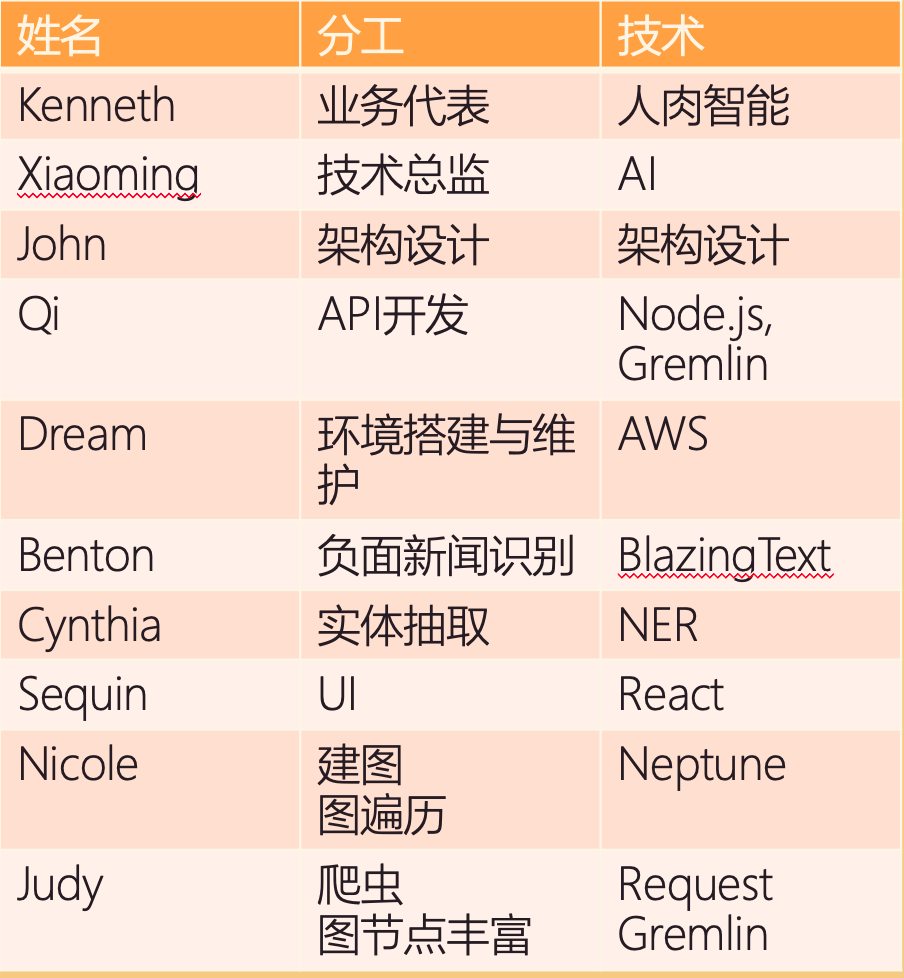## 评论